# Grouping

There is the Grouping section listed among view properties (except Calendar View), where you can specify the way how the displayed records will be grouped.

Grouping is quite simple and obvious; moreover, there are some additional options specifying the grouping. Such options depend on the type of column used for grouping.

If you select the Date or Formula-Date column, you can group by:

Equal Values, Day, Week, Month, Quarter, Year, Day in Month, Month in Year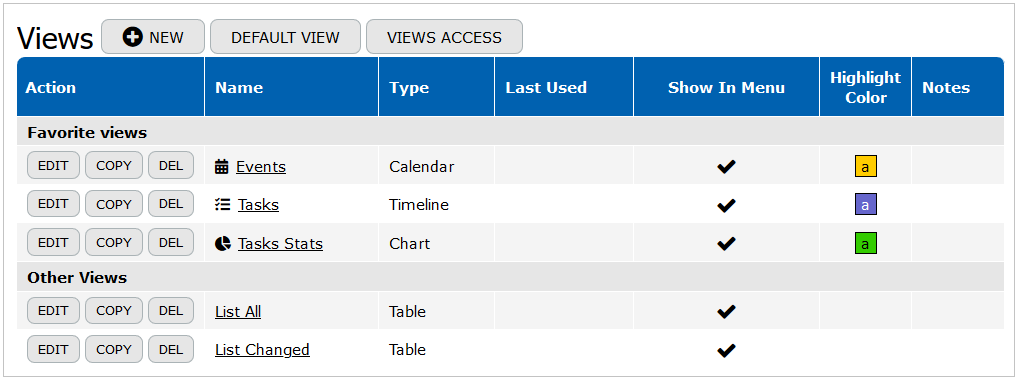If you choose the Time Column or Formula-Time, you can group by:

Equal Values, Second, Minute, HourIf you choose the Timestamp or Formula-Timestamp Column, you can group by:

Equal Values, Second, Minute, Hour, Day, Week, Month, Quarter, Year, Day in Month, Month in Year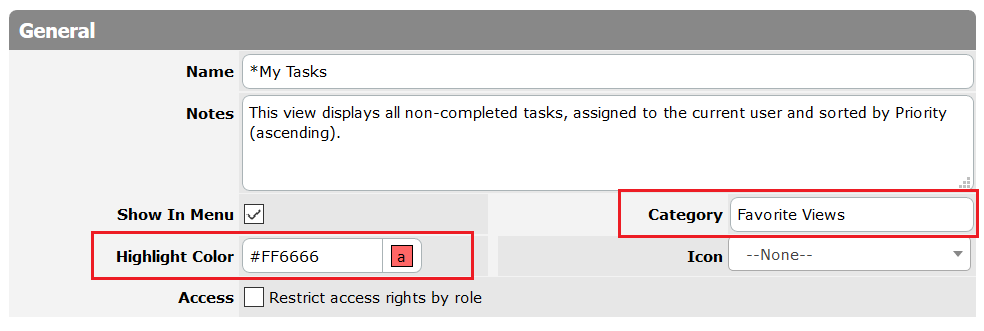If you choose the Duration Column or Formula-Duration, you can group by:

Equal Values, Second, Minute, Hour, Day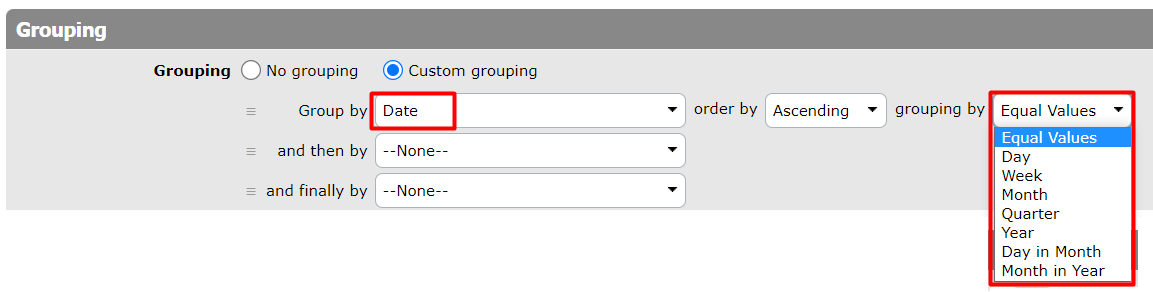If you choose the Numeric Column or Formula-Numeric, you can group by: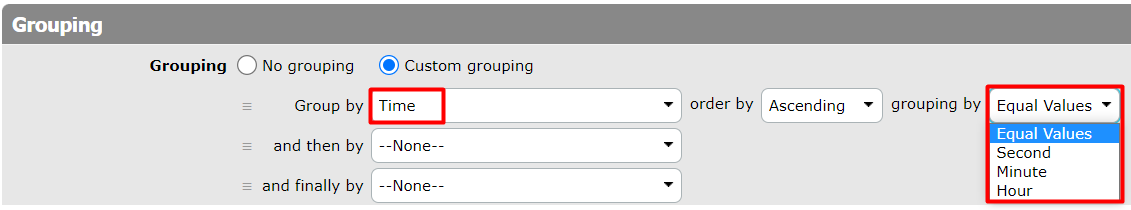If you choose the Text Column or Formula-Text, you can group by:

Equal Values, First Letter, First Word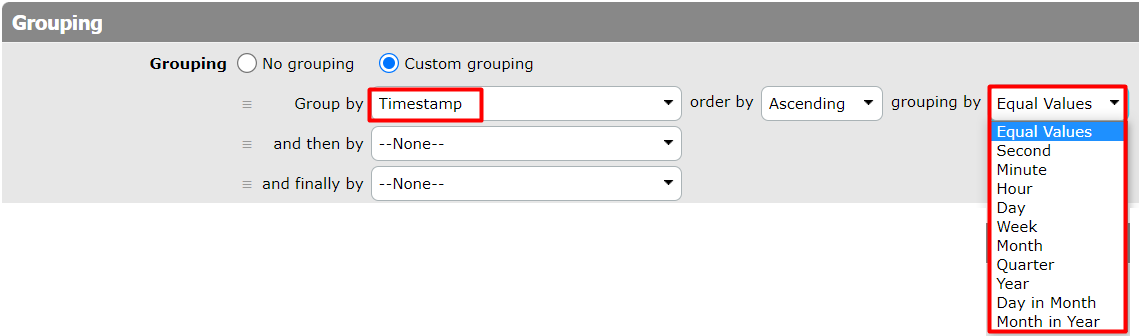Next: View Colorization